## 【Java源码解析】如何严谨地重写 equals 方法、getClass 方法与 instanceof 关键词用法比较-程序员宅基地

### 如何严谨地重写 equals 方法

#### 1 equals 方法概述

equals 方法我们都非常熟悉，equals 是 Object 基类中的模板方法 ，每个类中都有它的的存在，多数类或其抽象父类都以不同方式重写了 equals 方法。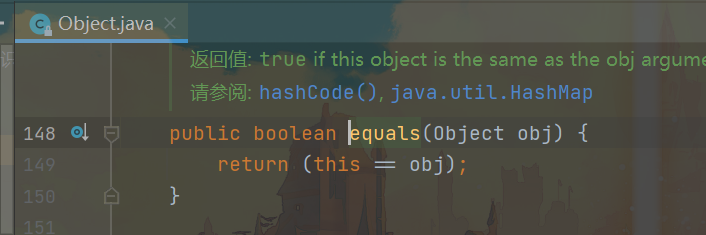#### 2 String 类中的 equals 方法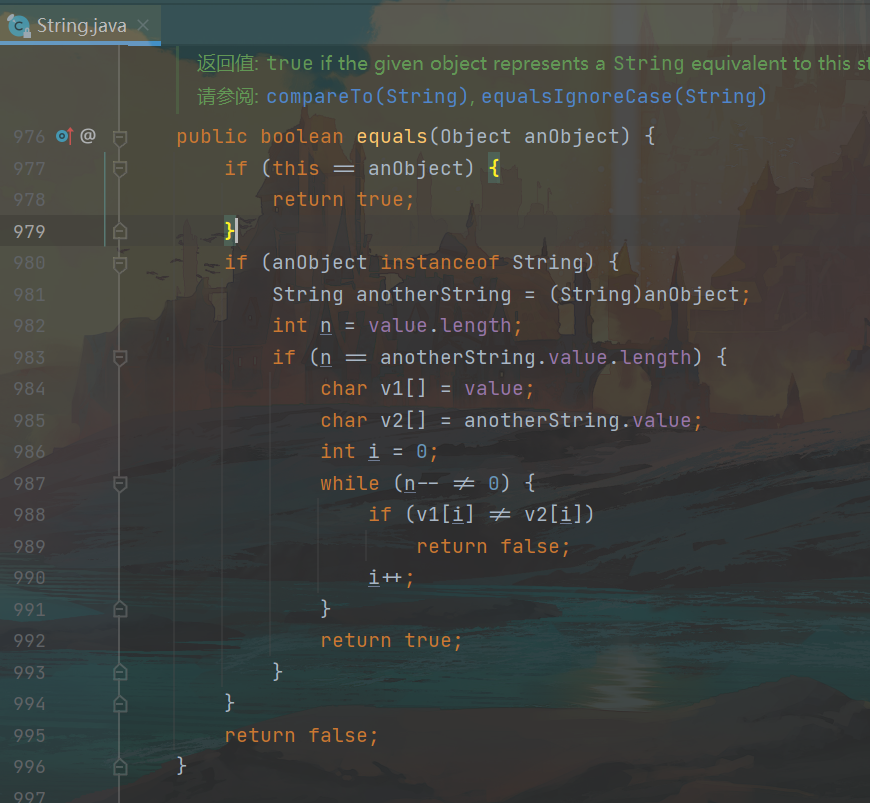#### 3 自定义 equals 方法时出现的问题

``````public class Test {

public static void main(String[] args) {

Person person1 = new Person("001");
Person person11 = new Person("001");
System.out.println("person1.equals(person11) = " + person1.equals(person11));

Student student1 = new Student("001", 100);
Student student11 = new Student("001", 100);
System.out.println("student1.equals(student11) = " + student1.equals(student11));

System.out.println("person1.equals(student1) = " + person1.equals(student1));
System.out.println("student1.equals(person1) = " + student1.equals(person1));
}
}

class Person {

private String idCard;

public Person() {

}

public Person(String name) {

this.idCard = name;
}

public String getIdCard() {

return idCard;
}

public void setIdCard(String name) {

this.idCard = name;
}

@Override
public boolean equals(Object obj) {

if (this == obj) {

return true;
}
if (obj instanceof Person) {
//obj为null也可以判断，这里不用单独处理
Person anotherPerson = (Person) obj;
return this.getIdCard().equals(anotherPerson.getIdCard());
}
return false;
}
}

class Student extends Person {

private Integer score;

public Student() {

}

public Student(String idCard, Integer score) {

super(idCard);
this.score = score;
}

public Integer getScore() {

return score;
}

public void setScore(Integer score) {

this.score = score;
}

@Override
public boolean equals(Object obj) {

if (!super.equals(obj)) {

return false;
}
if (obj instanceof Student) {

Student anotherStudent = (Student) obj;
return this.getScore() == anotherStudent.getScore();
}
return false;
}
}
``````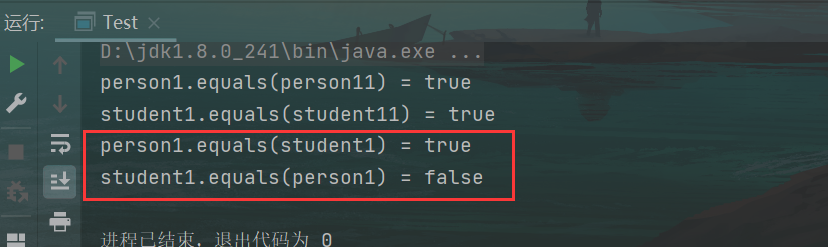#### 4 instanceof 关键词与 getClass 方法的比较

``````public class Test2 {

public static void main(String[] args) {

Person person = new Person();
System.out.println(person instanceof Student);

Student student = new Student();
System.out.println(student instanceof Person);
}
}

class Person {
}
class Student extends Person {
}
``````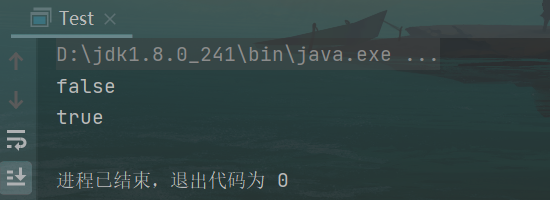``````public class Test3 {

public static void main(String[] args) {

Person person = new Person();
System.out.println(person.getClass());

Student student = new Student();
person = student;
System.out.println(person.getClass());
}
}

class Person {
}
class Student extends Person {
}
``````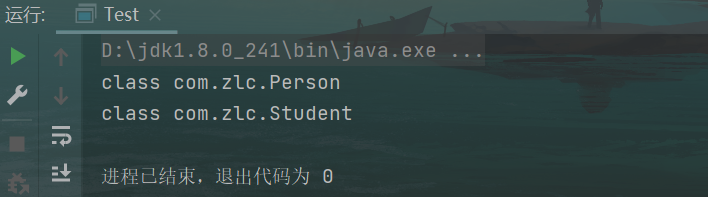#### 5 正确编写 equals 方法

Person 类

``````@Override
public boolean equals(Object obj) {

if (this == obj) {

return true;
}
if (getClass() == obj.getClass()) {
//obj为null也可以判断，这里不用单独处理
Person anotherPerson = (Person) obj;
return this.getIdCard().equals(anotherPerson.getIdCard());
}
return false;
}
``````

Student 类

``````@Override
public boolean equals(Object obj) {

if (!super.equals(obj)) {

return false;
}
if (getClass() == obj.getClass()) {

Student anotherStudent = (Student) obj;
return this.getScore() == anotherStudent.getScore();
}
return false;
}
``````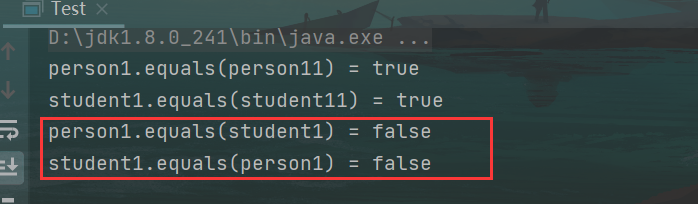### wordcount功能实现和单元测试-程序员宅基地

Github网址：https://gitee.com/ronanly/WordCount.git1.思路看到题目时，对于编写wordcount这个项目，首先想到它应该实现的功能有哪些，再来细化这些功能；我用的C语言，然后用gcc编译器来执行，在我的代码里，有以下功能实现函数：1.void countc(char *file) //返回文件的字符数2.void coun...

cadence SPB17.4 - allego - modify rename package pin number前言在做一个小板子的整体封装给底板用.焊盘都是随便放的, 没有编号.现在焊盘放完了, 想调整焊盘号码.先去官方本地文档, 官方论坛, 网上其他资料去查了一下, 讲的方法都是旧版的. 时间好早啊.并没有找到针对SPB17.4, 如何修改pin number的说明.笔记看旧版的方法都是改引脚的pin number TEXT, 试了一下, 可以改.在PCB Editor中新建或打_cadence封装修改引脚编号

### 整理收集的基于java、javaweb、jsp后台的2021年的几个微信小程序毕业设计毕设选题课题_黄菊华老师的博客-程序员宅基地

（1）Java微信小程序校园图书商城 大学生毕业设计教学视频https://ke.qq.com/course/3062230（2）大巴汽车票订票选座微信小程序（后台javaweb）源代码版https://ke.qq.com/course/3172321（3）电影院订票选座微信小程序（后台javaweb）源代码版https://ke.qq.com/course/3172320（4）微信小程序教室预约系统（后台javaweb）源代码版https://ke.qq.com/course/3172319